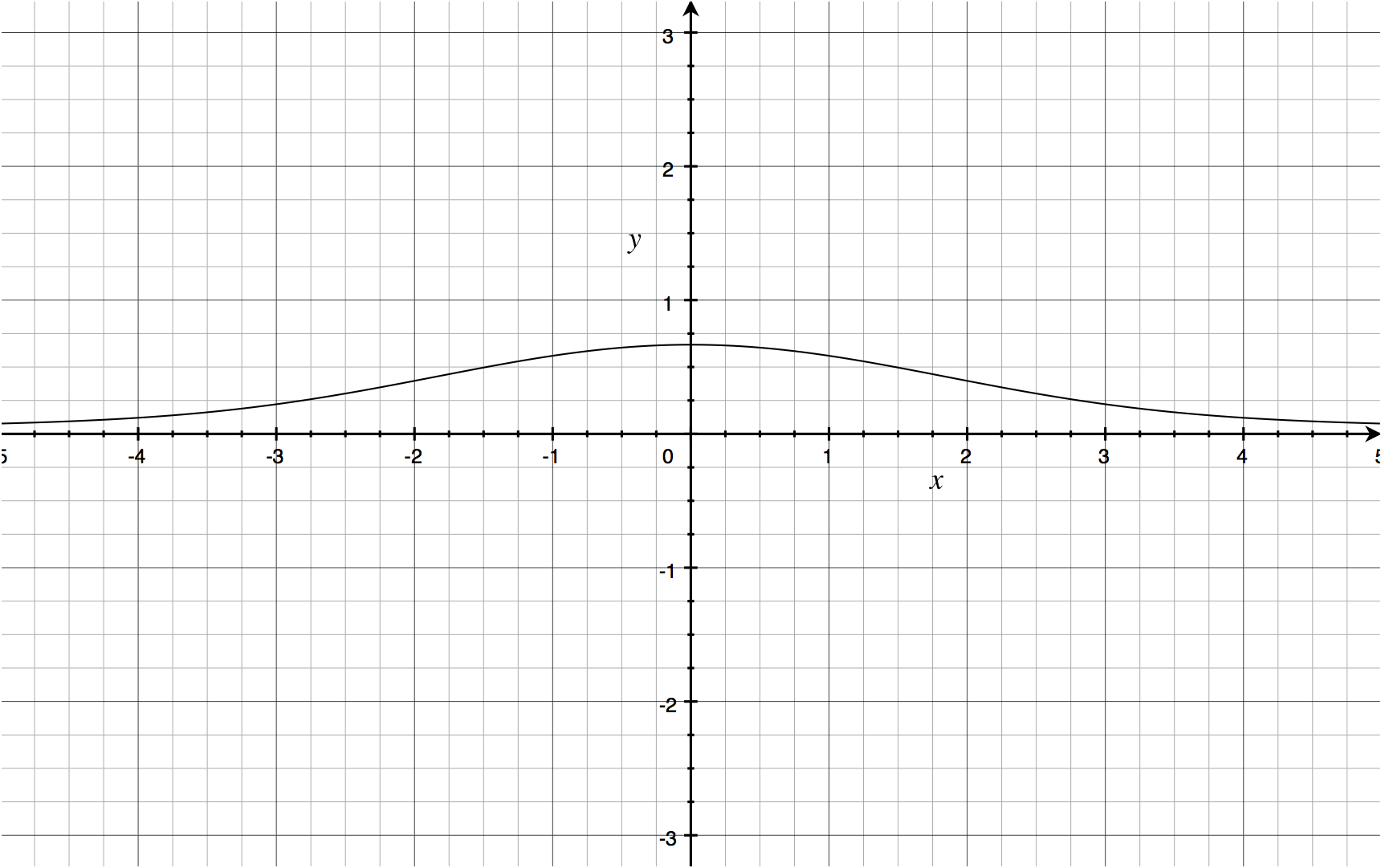# Limit of Impossibility

## 12 December 2013

This is a really cool trick I only recently learned. Evaluating limits has useful applications, and this little trick allows the evaluation of limits which, on the surface, seem impossible. Consider

$\lim_{x\to 0}\frac{2\cos 2x-2+4x^2}{2x^4}.$

The denominator poses a problem, as $\lim_{x\to 0}2x^4 = 0$, therefore the entire limit is of the form $\frac{-2}{0}$, which suffice it to say is not acceptable. It isn’t one of the forms to which L’Hôpital’s rule1 applies.

We expect the limit to be evaluable, however, by inspecting the graph.However, there is another way to evaluate this limit, though it involves making it appear very complex. By expanding $\cos 2x$ using the Maclaurin series for $\cos x$, the limit can be expanded.

$\begin{array}{rl} & \lim_{x\to 0}\frac{ 2\sum_{k=0}^{\infty}\frac{(-1)^k(2x)^{2k}}{(2k)!}-2+4x^2 }{2x^4} \\ =& \lim_{x\to 0}\frac{ 4x^2-2+ 2\left[ 1- \frac{(2x)^2}{2!}+ \frac{(2x)^4}{4!}- \frac{(2x)^6}{6!}+ \cdots \right]}{2x^4} \\ =& \lim_{x\to 0}\frac{ 4x^2 -2+ 2- \frac{2(2x)^2}{2!}+ \frac{2(2x)^4}{4!}- \frac{2(2x)^6}{6!}+ \cdots }{2x^4} \\ =& \lim_{x\to 0}\frac{ \frac{2(2x)^4}{4!}- \frac{2(2x)^6}{6!}+ \cdots }{2x^4} \\ =& \lim_{x\to 0}\frac{ 2x^4\cdot\left[ \frac{2^4}{4!}- \frac{2^6x^2}{6!}+ \frac{2^8x^4}{8!}- \cdots \right]}{2x^4} \\ =& \frac{16}{24}=\frac{2}{3} \\ \end{array}$

The remaining terms from the Maclaurin series are eliminated because they tend to zero when the limit is evaluated, which leaves us with the expected value of $\frac{2}{3}$. That is unqualifiedly cool.

This is a solution for §9.4 Exercise #9 from Calculus: Early Transcendentals.